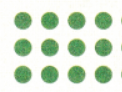# McGraw Hill Math Grade 3 Chapter 5 Lesson 9 Answer Key Problem Solving

## McGraw-Hill Math Grade 3 Answer Key Chapter 5 Lesson 9 Problem Solving

Solve

Decide which facts you need. Then solve.

Question 1.
Mr. Williams arranges his class into 3 groups for story time. There are 5 students in each group. Each group reads 4 books. How many students are in Mr. Williams’s class?
15 books

Explanation:
Mr. Williams arranges his class into 3 groups for story time
There are 5 students in each group
We need to find the number of children in Mr. William’s class
Multiply to find
3 groups x 5 students = 15 students
So, thee are 15 students in Mr. William’s class.

Question 2.
Luis is putting stickers in his journal. Each sticker is 1 inch long. He sets them in 3 rows of 4. How many stickers does Luis have? Write a division equation for this problem and solve.